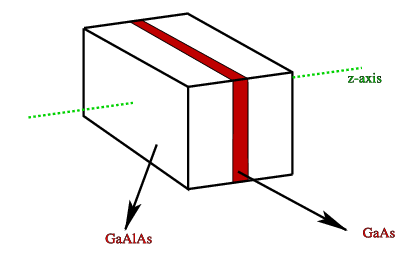# 4.6: A physical system (approximately) described by a square well

$$\newcommand{\vecs}{\overset { \rightharpoonup} {\mathbf{#1}} }$$ $$\newcommand{\vecd}{\overset{-\!-\!\rightharpoonup}{\vphantom{a}\smash {#1}}}$$$$\newcommand{\id}{\mathrm{id}}$$ $$\newcommand{\Span}{\mathrm{span}}$$ $$\newcommand{\kernel}{\mathrm{null}\,}$$ $$\newcommand{\range}{\mathrm{range}\,}$$ $$\newcommand{\RealPart}{\mathrm{Re}}$$ $$\newcommand{\ImaginaryPart}{\mathrm{Im}}$$ $$\newcommand{\Argument}{\mathrm{Arg}}$$ $$\newcommand{\norm}{\| #1 \|}$$ $$\newcommand{\inner}{\langle #1, #2 \rangle}$$ $$\newcommand{\Span}{\mathrm{span}}$$ $$\newcommand{\id}{\mathrm{id}}$$ $$\newcommand{\Span}{\mathrm{span}}$$ $$\newcommand{\kernel}{\mathrm{null}\,}$$ $$\newcommand{\range}{\mathrm{range}\,}$$ $$\newcommand{\RealPart}{\mathrm{Re}}$$ $$\newcommand{\ImaginaryPart}{\mathrm{Im}}$$ $$\newcommand{\Argument}{\mathrm{Arg}}$$ $$\newcommand{\norm}{\| #1 \|}$$ $$\newcommand{\inner}{\langle #1, #2 \rangle}$$ $$\newcommand{\Span}{\mathrm{span}}$$$$\newcommand{\AA}{\unicode[.8,0]{x212B}}$$

After all this tedious algebra, let us look at a possible physical realization of such a system. In order to do that, I shall have to talk a little bit about semi-conductors. A semiconductor is a quantum system where the so-called valence electrons completely fill a valence band, and are separated by a gap from a set of free states in a conduction band. These can both be thought of a continuous set of quantum states. The energy difference between the valence and conduction bands is different for different semi-conductors. This can be used in so-called quantum-well structures, where we sandwich a thin layer of, e.g., $$\ce{GaAs}$$ between very thick layers of $$\ce{GaAlAs}$$ (Figure $$\PageIndex{1}$$).Figure $$\PageIndex{1}$$: A schematic representation of a quantum well

Since the gap energy is a lot smaller for GaAs than for GaAlAs, we get the effect of a small square well (in both valence and conduction bands). The fact that we can have a few occupied additional levels in the valence, and a few empty levels in the conduction band can be measured.Figure $$\PageIndex{2}$$:

The best way to do this, is to shine light on these systems, and see for which frequency we can create a transition (just like in atoms).

This page titled 4.6: A physical system (approximately) described by a square well is shared under a CC BY-NC-SA 2.0 license and was authored, remixed, and/or curated by Niels Walet via source content that was edited to the style and standards of the LibreTexts platform; a detailed edit history is available upon request.